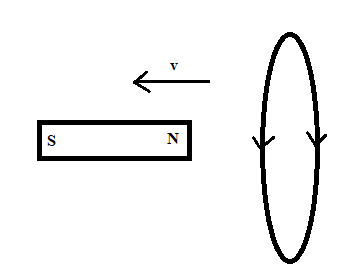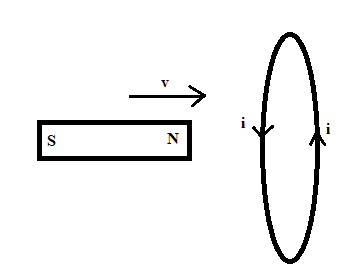# A bar magnet is positioned near a coil of wire horizontally. What is the direction of the current...

## Question:

A bar magnet is positioned near a coil of wire horizontally. What is the direction of the current in the resistor when the magnet is moved (a) to the left and (b) to the right?

## Lenz's Law

Lenz's Law is very useful in determining the direction of induced current during the electromagnetic induction, according to Lenz's law the direction of the induced current should be such that it opposes the cause producing it, i.e. the change in the magnetic flux linked with a closed loop.

Part A)As the magnet is moving towards the Left i.e away from the loop as shown in the diagram belowso the magnetic flux linked with the coil decreases, and according to Lenz's Law, the direction of the induced current should be clockwise so that induced current can increase the decreasing magnetic flux.

Part B) As the magnet is moving towards the right i.e towards the loop as shown in the diagram belowso the magnetic flux linked with the coil increases, and according to Lenz's Law the direction of the induced current should be anticlockwise so that induced current can reduce the increasing magnetic flux.